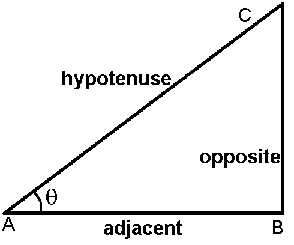# Triangle Angles and Side Calculation Online

Please select the option and enter required values then press calculate button:

 I want to calculate Angle Opposite Side Hypotenuse Side Adjacent Side and Angle Opposite Side Hypotenuse Side Adjacent Side

 Opposite Side : Hypotenuse Side : Adjacent Side : Angle q :[Max 90] Angle b : (90-q) =

Important Formula:

Sin(q) = Opposite / Hypotenuse

Cos(q) = Adjacent / Hypotenuse

Tan(q) = Opposite / Adjacent

Select what (angle / sides) you want to calculate, then enter the values in the respective rows and click calculate.

If you want to calculate hypotenuse enter the values for other sides and angle.